# pylops.Restriction#

class pylops.Restriction(dims, iava, axis=-1, inplace=True, forceflat=None, dtype='float64', name='R')[source]#

Restriction (or sampling) operator.

Extract subset of values from input vector at locations iava in forward mode and place those values at locations iava in an otherwise zero vector in adjoint mode.

Parameters
dims

Number of samples for each dimension

iava

Integer indices of available samples for data selection.

axisint, optional

New in version 2.0.0.

Axis along which restriction is applied to model.

inplacebool, optional

Work inplace (True) or make a new copy (False). By default, data is a reference to the model (in forward) and model is a reference to the data (in adjoint).

forceflatbool, optional

New in version 2.2.0.

Force an array to be flattened after rmatvec. Note that this is only required when len(dims)=2, otherwise pylops will detect whether to return a 1d or nd array.

dtypestr, optional

Type of elements in input array.

namestr, optional

New in version 2.0.0.

Name of operator (to be used by pylops.utils.describe.describe)

pylops.signalprocessing.Interp

Interpolation operator

Notes

Extraction (or sampling) of a subset of $$N$$ values at locations iava from an input (or model) vector $$\mathbf{x}$$ of size $$M$$ can be expressed as:

$y_i = x_{l_i} \quad \forall i=0,1,\ldots,N-1$

where $$\mathbf{l}=[l_0, l_1,\ldots, l_{N-1}]$$ is a vector containing the indices of the original array at which samples are taken.

Conversely, in adjoint mode the available values in the data vector $$\mathbf{y}$$ are placed at locations $$\mathbf{l}=[l_0, l_1,\ldots, l_{M-1}]$$ in the model vector:

$x_{l_i} = y_i \quad \forall i=0,1,\ldots,N-1$

and $$x_{j}=0$$ for $$j \neq l_i$$ (i.e., at all other locations in input vector).

Attributes
shapetuple

Operator shape

explicitbool

Operator contains a matrix that can be solved explicitly (True) or not (False)

Methods

 __init__(dims, iava[, axis, inplace, ...]) adjoint() apply_columns(cols) Apply subset of columns of operator cond([uselobpcg]) Condition number of linear operator. conj() Complex conjugate operator div(y[, niter, densesolver]) Solve the linear problem $$\mathbf{y}=\mathbf{A}\mathbf{x}$$. dot(x) Matrix-matrix or matrix-vector multiplication. eigs([neigs, symmetric, niter, uselobpcg]) Most significant eigenvalues of linear operator. mask(x) Apply mask to input signal returning a signal of same size with values at iava locations and 0 at other locations matmat(X) Matrix-matrix multiplication. matvec(x) Matrix-vector multiplication. reset_count() Reset counters rmatmat(X) Matrix-matrix multiplication. rmatvec(x) Adjoint matrix-vector multiplication. todense([backend]) Return dense matrix. toimag([forw, adj]) Imag operator toreal([forw, adj]) Real operator tosparse() Return sparse matrix. trace([neval, method, backend]) Trace of linear operator. transpose()

## Examples using pylops.Restriction#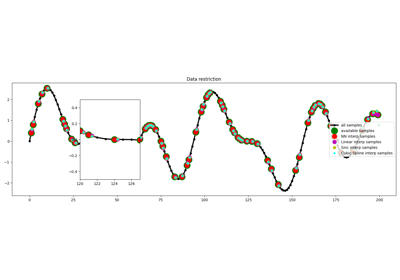Restriction and Interpolation

Restriction and Interpolation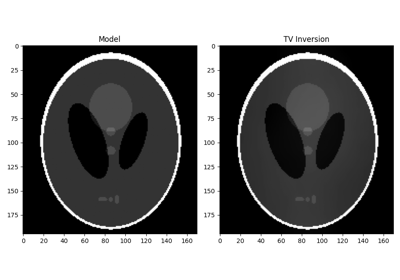Total Variation (TV) Regularization

Total Variation (TV) Regularization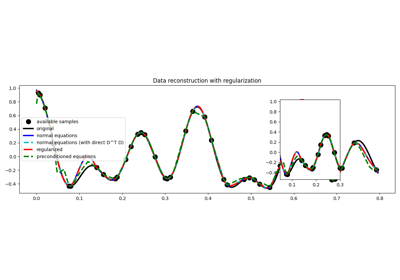03. Solvers

03. Solvers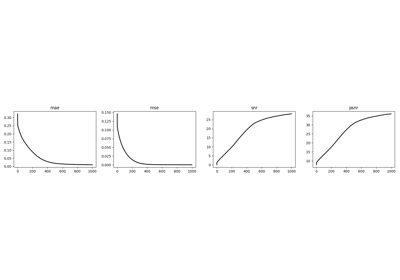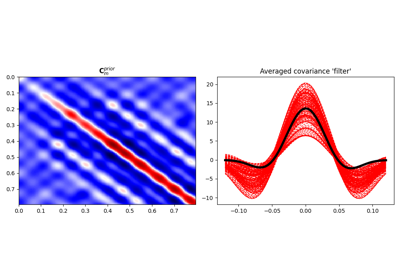04. Bayesian Inversion

04. Bayesian Inversion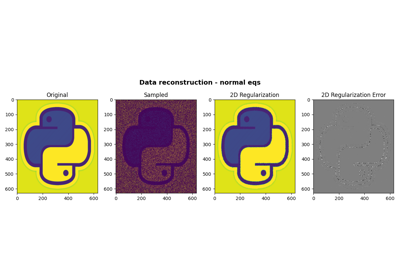06. 2D Interpolation

06. 2D Interpolation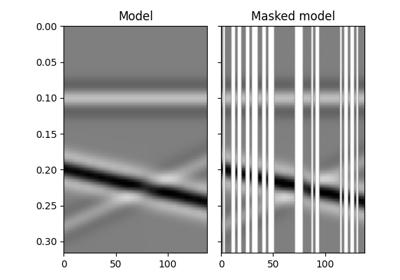12. Seismic regularization

12. Seismic regularization